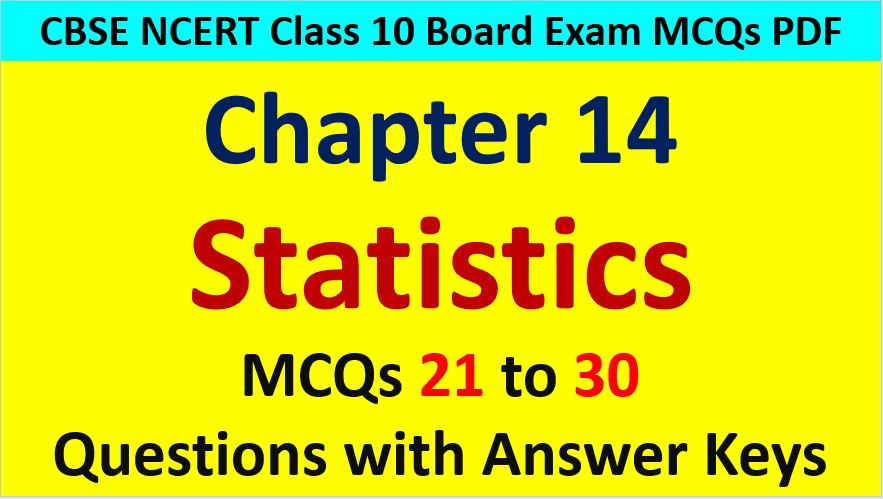Saturday, December 4, 2021
Home > CBSE Class 10 > Important MCQ Questions for Class 10 Maths Chapter 14 Statistics

# Important MCQ Questions for Class 10 Maths Chapter 14 StatisticsHi students, Welcome to AMBiPi (Amans Maths Blogs). In this article, you will get Important MCQ Questions for Class 10 Maths Chapter 14 Statistics. You can download this PDF and save it in your mobile device or laptop etc.

Important MCQ Questions of Statistics Class 10 Question No 21:

The sum of 12 observations is 600, then their mean is

Option A : 30

Option B : 40

Option C : 50

Option D : 60

Option C : 50

Important MCQ Questions of Statistics Class 10 Question No 22:

The mean of cubes of first 15 natural numbers is

Option A : 980

Option B : 960

Option C : 1920

Option D : 1940

Option B : 960

Important MCQ Questions of Statistics Class 10 Question No 23:

The mean of x, y, z is y, then x + z = ?

Option A : y

Option B : 3y

Option C : 2y

Option D : 4y

Option C : 2y

Important MCQ Questions of Statistics Class 10 Question No 24:

The median of the first 16 prime numbers is

Option A : 12

Option B : 24

Option C : 21

Option D : 30

Option C : 21

Important MCQ Questions of Statistics Class 10 Question No 25:

If the mean of a set of 15 observation is 12 and that of another set of 10 observation is 15, then find the mean of the combined set is

Option A : 15

Option B : 14.1

Option C : 12.5

Option D : 13.2

Option D : 13.2

Important MCQ Questions of Statistics Class 10 Question No 26:

The mean of 20 observations was found to be 40. It was later discovered that 25 and 36 were misread as 23 and 42, then find the correct mean.

Option A : 41

Option B : 39

Option C : 38.8

Option D : 39.8

Option D : 39.8

Important MCQ Questions of Statistics Class 10 Question No 27:

The mean of the data x, x+a, x+2a, x+3a,… to (2n+1) terms, is

Option A : (x + an)

Option B : (x + an) / n

Option C : (x + an) / 2

Option D : (x + an) / (2n + 1)

Option A : (x + an)

Important MCQ Questions of Statistics Class 10 Question No 28:

If the difference between the mode and the median is 36, then find the difference between the median and the mean.

Option A : 16

Option B : 33

Option C : 18

Option D : 32

Option C : 18

Important MCQ Questions of Statistics Class 10 Question No 29:

The lower limit and the length of an exclusive class interval are 15 and 25 respectively. Then, the class mark is

Option A : 26.5

Option B : 27.5

Option C : 28

Option D : 27

Option B : 27.5

Important MCQ Questions of Statistics Class 10 Question No 30:

2(Median – Mean) = Mode – ______

Option A : Mean

Option B : 2 Mean

Option C : Median

Option D : 3 Median

Option C : Median

CBSE Class 10 Maths Chapterwise MCQs
1 : Real Numbers MCQ
| 1 to 10 | 11 to 20 | 21 to 30 | 31 to 40 | 41 to 50
2 : Polynomials MCQ
| 1 to 10 | 11 to 20 | 21 to 30 | 31 to 40 | 41 to 50
3 : Linear Equations MCQ
| 1 to 10 | 11 to 20 | 21 to 30 | 31 to 40 | 41 to 50
| 1 to 10 | 11 to 20 | 21 to 30 | 31 to 40 | 41 to 50
5 : Arithmetic Progression MCQ
| 1 to 10 | 11 to 20 | 21 to 30 | 31 to 40 | 41 to 50
6 : Triangles MCQ
| 1 to 10 | 11 to 20 | 21 to 30 | 31 to 40 | 41 to 50
7 : Coordinate Geometry MCQ
| 1 to 10 | 11 to 20 | 21 to 30 | 31 to 40 | 41 to 50
8 : Introduction to Trigonometry MCQ
| 1 to 10 | 11 to 20 | 21 to 30 | 31 to 40 | 41 to 50
9 : Application of Trigonometry MCQ
| 1 to 10 | 11 to 20 | 21 to 30 | 31 to 40 | 41 to 50
10 : Circles MCQ
| 1 to 10 | 11 to 20 | 21 to 30 | 31 to 40 | 41 to 50
11 : Constructions MCQ
| 1 to 10 | 11 to 20
12 : Area Related to Circles MCQ
| 1 to 10 | 11 to 20 | 21 to 30 | 31 to 40 | 41 to 50
13 : Surface Area & Volume MCQ
| 1 to 10 | 11 to 20 | 21 to 30 | 31 to 40 | 41 to 50
14 : Statistics MCQ
| 1 to 10 | 11 to 20 | 21 to 30 | 31 to 40 | 41 to 50
15 : Probability MCQ
| 1 to 10 | 11 to 20 | 21 to 30 | 31 to 40 | 41 to 50
16 : Previous Year Maths MCQs from CBSE Board Class 10
| 1 to 100
AMBiPi
error: Content is protected !!